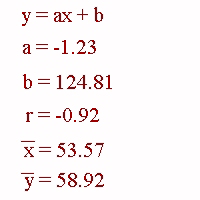### CFA Practice Question

There are 208 practice questions for this study session.

### CFA Practice Question

If you run linear regression on a set x-y data and obtain the results shown below then the percentage of variation in variable y due the variable x is ______.A. -92%
B. 92%
C. 84.64%